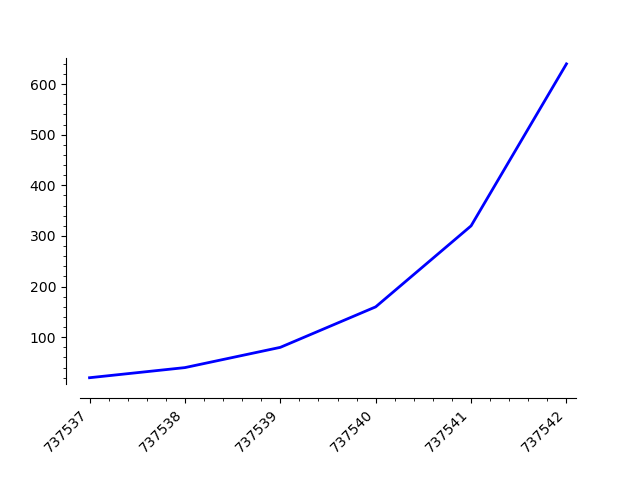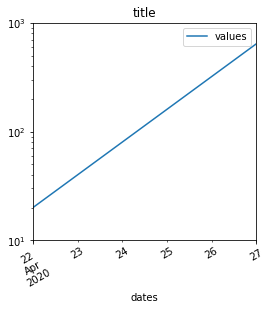Ask Your Question

# How to change ticks labels attributes for the 2D plot?

Is there a way to access ticks labels to rotate them? I've tried Graphics.matplotlib() and set_rotation(), but this doesn't seem to produce changes. Am I doing wrong things? In the example below the formatter and locator are working correctly, but the problem is all labels are oriented horizontally, messing all together. Need to rotate them.

import csv
from datetime import datetime
from matplotlib import ticker
from matplotlib import dates

data = [ ( '04/22/20', '04/23/20', '04/24/20','04/25/20','04/26/20', '04/27/20' ), (20, 40, 80, 160, 320, 640) ]
labels = data
labels = map(lambda x: dates.date2num(datetime.strptime(x, '%m/%d/%y')), labels)
labels = list(labels)
values = data
values = map(lambda x: int(x), values)

# Z is a list of [(x1,y1),(x2,y2)...]
# x1, x2, ... are dates
# y1, y2, ... are values
Z = zip(labels, values)
Z = list(Z)

p = list_plot( Z, ticks=[1, None], tick_formatter=[dates.DateFormatter('%d.%m.%Y'), None], axes_labels=[ 'Days', '$\\log \\;{N}$' ], plotjoined=true, thickness=2, figsize=4, scale='semilogy' )

G = p.matplotlib()
labels = G.axes.xaxis.get_ticklabels()
labels = list(labels)

for label in labels:
label.set_rotation(45)

p


This outputs the plot with an ugly x-axis on which all the dates are messed up. How to fix that?edit retag close merge delete

## Comments

A minimal example that can by copy-pasted in a fresh Sage session helps others get started on an answering a question, therefore increasing the chances of getting an answer. In this case,

• include the appropriate import statements so that dates.DateFormatter will work
• provide an actual Z (with just enough dates to illustrate the problem)

Hello, thank you for your response. I've added the information needed to comply.

Following what is explained in this link, it seems the following should work:

sage: figure = p.matplotlib()
sage: figure.autofmt_xdate(rotation=45)
sage: figure.savefig('a.png')


but the last line returns an error because figure.canvas is None

Yes, thanks for this answer. I've already tried that with the same result. But this example is for pure matplotlib, not the sage Graphics object. I would like to know, what does that matplotlib() exactly do? I even tried:

from matplotlib.pyplot import figure
...
fig = figure()
fig = p.matplotlib(fig)
...


to draw the plot on the new figure provided, but this doesn't work for me. So, the question is, is this matplotlib() function supposed to give the access to p attributes, which were previosly set when the plot was created, and should the changes made on the figure effect onto the plot? And is the plot is supposed to be redrawn on the new figure when the plot is passed as an argument to matplotlib()?

Suggestion: format dates following the international standard ISO 8601.

## 2 Answers

Sort by » oldest newest most voted

Hello, @MrDvbnhbq! I can see that you already got an answer for your question. However, I have an alternative approach.

As far as I know, there is no direct way to do this in SageMath, so we are going to use a mix of Sage plots and Matplotlib plots, i.e., we will use the matplotlib() command. All the arguments that you passed to list_plot() can be passed to the matplotlib() command, except for the thickness and the plotjoined parameters, which are exclusive of the former.

Consider the following instructions:

%matplotlib inline
import csv
from datetime import datetime
from matplotlib import ticker
from matplotlib import dates
import matplotlib.pyplot as plt

data = [ ( '04/22/20', '04/23/20', '04/24/20','04/25/20','04/26/20', '04/27/20' ), (20, 40, 80, 160, 320, 640) ]
labels = data
labels = map(lambda x: dates.date2num(datetime.strptime(x, '%m/%d/%y')), labels)
labels = list(labels)
values = data
values = map(lambda x: int(x), values)

Z = zip(labels, values)
Z = list(Z)

p = list_plot(Z, plotjoined=true, thickness=2)

G = p.matplotlib(figure=plt.gcf(),scale='semilogy',tick_formatter=[dates.DateFormatter('%d.%m.%Y'), None], axes_labels=[ 'Days', '$\\log \\;{N}$' ], ticks=[1, None], figsize=4)
G.autofmt_xdate(rotation=45)
G.show()


I have made only three changes to your code:

1. I have used the magic %matplotlib inline as the first line, so that Matplotlib figures are shown in SageCell or Jupyter(lab).
2. I have imported pyplot under the name plt on line 6.
3. I have moved all the arguments you used in list_plot to the matplotlib() command, including the additional argument figure=plt.gcf().
4. Concerning the rotation of the xticks, I used the G.autofmt_xdate(rotation=45) command. However, you can also use the plt.xticks(rotation=45) instruction; since Matplotlib already knows you are working with the current figure (that's the plt.gcf()), it will apply the changes directly to it, in that case.

That's it!

By the way, you can remove the G.show() line, which I included for completeness.

The problem with your previous approach, and @Sébastien's is that the matplotlib() command does not preserve the semilog y-axis, nor the ticks formatter, etc. So, instead of using those options in list_plot where they will be overwritten later by matplotlib(), I passed them to the latter, so it can't ignore them.

The final result should look like this:I hope this helps!

more

## Comments

Looks good ! The interface between Sage and the underlying matplotlib, and the possilibty go work on that backend should be clarified and polished. Do you have an idea why some options passed to Sage (e.g. semilog y-axis) are not transfered to matplotlib ?

2

Also, since you seem at ease with the graphic internals, did you consider helping in improving that part of Sage ?

Hello, @tmonteil! As for your first question, yes, I know why some options passed to Sage are not transferred to Matplotlib. The reason is that the matplotlib() command has some of the same arguments as the list_plot command, and those have default values, which are not overwritten by the Sage plot properties.

Let me give you an example. Suppose you use the following command:

q = p.matplotlib()


where p is a plot. If you check the signature of this method, you will notice it has the argument axes with default value None. As expected, when you don't use the axes argument here, it assumes the value None. However, the following that should happen in this case is that the code should use the axes value set for p, but That doesn't happen!

It should be easy to fix.

2

Continuing with your second question, @tmonteil, yes, I would be happy to help improving the Sage-Matplotlib interface. Actually, I've been thinking this for a long time. There is also much to improve in the 3d plots capabilities (check, for example, this question).

The following two weeks I am going to be very busy, but then I can start adding some improvements. Is there a recommended way to proceed? Should I work freely or should I pass every decision through the sage-devel forum?

A discussion on sage-devel is needed when two persons do not agree on a decision and they ask on sage-devel for comments to get some consensus. In your case, you propose a clear improvement so such a discussion will not be needed. I suggest you to create a ticket, post a branch and reviewers will check it.

Ok, so it seems what was missing is the "get current graphics", see this other question. Now this works:

sage: import matplotlib.pyplot as mpl
sage: figure = p.matplotlib(figure=mpl.gcf())
sage: figure.autofmt_xdate(rotation=45)
sage: figure.show()
sage: figure.savefig('a.png')But then, the dates and log y scales need more work. There must be a way to fix this... Alternatively, you may use pandas (which is an optional python library that one can install by doing sage -pip install pandas):

sage: from datetime import datetime
sage: data = [ ( '04/22/20', '04/23/20', '04/24/20','04/25/20','04/26/20', '04/27/20'), (20, 40, 80,160, 320, 640) ]
sage: labels = [datetime.strptime(x, '%m/%d/%y') for x in data]
sage: values = [int(a) for a in data]
sage: import pandas as pd
sage: df = pd.DataFrame({'dates':labels,'values':values})
sage: df
dates  values
0 2020-04-22      20
1 2020-04-23      40
2 2020-04-24      80
3 2020-04-25     160
4 2020-04-26     320
5 2020-04-27     640
sage: df.plot(x='dates', y='values', title='title', rot=30, logy=True, figsize=[4,4], yticks=[10,100,1000])For the image to appear in a Jupyter notebook, add the following in the first cell:

%matplotlib inline

more

## Comments

Aha!.. That's a pretty nice solution. Will definitely dig deeper into pandas too. Thanks a lot for your help! Cheers 👍

## Your Answer

Please start posting anonymously - your entry will be published after you log in or create a new account.

Add Answer

## Stats

Asked: 2020-04-27 20:59:05 +0200

Seen: 232 times

Last updated: May 25 '20